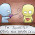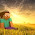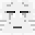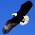## Tuesday, 5 March 2013

### Algebra... Different "Faces" of Algebra (II)

The perimeter of a rectangle is (6x + 5y) cm.
Suggest 2 possible dimensions of the rectangle.

e.g.
Length of rectangle = 2x + 2y cm
Breadth of rectangle = x + 0.5y cm
Check:
Perimeter = 2 (2x + 2y) + 2 (x + 0.5y) cm
= 4x + 4y + 2x + y cm
= 6x + 5y cm

1.length - 3x cm
breath - 2.5y cm

2.2X+Y, X+3/2Y

3.3Xcm is the length
2.5ycm is the breadth

4.Length of rectangle = (1.25x + 2.5y) cm
Breadth of rectangle = 1.25 cm

5.1)
Length=(3x+1.5y)cm
Total=(6x+5y) cm
2)
Length=(2x+2.5y)cm
Total=(6x+5y)cm

6.length-2.5y
breath-3x

1.the - is a dash not a negative sign

7.Length = 3x cm
Breadth = 2.5y cm

8.The length of the rectangle is 3x cm.
The breadth of the rectangle is 2.5y cm.

9.1 (x+2.5y)(2x)
2 (2x+y)(x+3y)

10.There are many possibilities.One is : Length: 3x

11.length: 2.5x cm
breadth: 0.5x + 2.5y cm

Perimeter= (2.5x cm)+(2.5x cm)+(0.5+2.5y cm)+(0.5+2.5y cm)
= ( 6x + 5y ) cm

1.This comment has been removed by the author.

2.12.Length-(1x+2y) cm
Check- 2(1x+2y) cm + 2(2x+0.5) cm = (6x+5y) cm

13.length : [1.5y + 3x]cm
breadth : 1y cm

14.This comment has been removed by the author.

1.This comment has been removed by the author.

15.length: (3x+0.5y)
breath: (2y)cm
Check: 2(3x+0.5y)cm + (2y)cm=(6x+5y)

16.length-(2x+y)cm
total-2(2x+y)cm+2(x+1.5)cm=(6x+5y)cm

17.length = 1.5x cm
breadth = 1.25y cm

18.Length:2.5y

19.length: 3x cm
breath: 2.5y cm

20.1)Length : (1.5x + 1.25y)
1)Breadth : (1.5x + 1.25y)
1) Perimeter : 1.5x + 1.5x + 1.5x +1.5x + 1.25y + 1.25y + 1.25y + 1.25y = 6x + 5y

2)Length : (3x)
21.